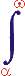MYSLENE DREVO We build the ukrainian Ukraine!

 ? Mail to site News feed (RSS)

# 1.6. Examples of the models and real closed systems

V. I. Melnikov

The structural and functional analyses of some of models and real CCS are shown in the table 1. The most close to the ACS are the CCS the stable balance of which is annihilation. The division “object – anti-object (the environment)” is relative (arbitrary) in many cases especially for the integral parts of the CCS. As a rule, exactly for those systems such a division was not made up historically.

Some of the examples till now have no fixed parameters (they are marked by the “?”). Most of them are in the most simple and complicated CCS. These deficiencies in the system overview mean the lack of investigations in it, as well as the possible investigation trend. For the model it could mean an imperfection. The shown systems are not neither simple nor complicated from the TCS positions, but their parameters are not described by the adopted quality and quantity indexes and the systems of measure units. The main parameters shown below are unified for any systems and objects. The methodologically depended specific parameters are defined by the object nature.

For example, balance as the final aim of the interaction is the unified parameter of any СCS. But such a variety as mobile balance of a system person-environment (with metabolism, information and energy exchange etc.) is peculiar only for this system. In the same way we can analyze a person’s subsystems of any hierarchical level, i.e. separate organs, parts of organs etc.

For the complicated compound systems, that consist of many objects, have different states (not only those in concentrated, but in distributed states (environment) as well) and those that have some of assumptions that are qualified as independent functioning factors (i.e. states), the more convenient description as an analytic one.

The analytically described concept for the cases of easy and complicated ACS and СCS is given below .

The easiest case of ACS as it was said above could be given in a form of two opposite parts: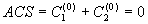= R0, (8)

where С1(0), С2(0) – the state of the first and the second parts relative to absolute zero (ACS); R(0) – corresponding level of equality (absolute equality).

If ACS consists of many parts we cam write: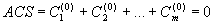= R(0), (9)

Taking into account that some of the parts of an ACS are integral (objects) and the other are not (environments), we would write: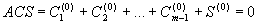= R(0)

where S(0) – the summary state of all not integral parts of an ACS (environment).

The CCS could be defined through the ACS in a following way: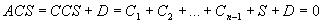= R(0)

where C1, C2 etc. are approximate states of objects of an ACS; S – approximate state of an environment; D – a summary (state) of taken assumptions

or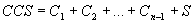.

Taking into account possible levels of interaction and balances for the first level we could write: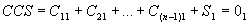= R(0)1

where C11, C21 etc. is an approximate state of the first level of a first, second etc. objects correspondingly; S1 is an approximate state of an environment of the first level of interaction; R(0)1 is an approximate balance of the first level; 01 is the summary state of a RCS of the first level: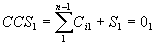~ R(0)1 (10)

or for the second level: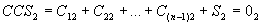~ R(0)2,

or: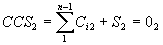~ R(0)2.

For the “m” level: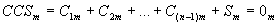~ R(0)m,

or: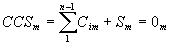~ R(0)m (11)

Summing up all of the parts of an ACS on all levels and turning to a RCS we will write: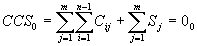(12)

where Cij is the state of a CCS’s parts of an object “i” of a “j” level; Sj is a state of the level’s environments of a “j” level; 00 is a summary state of a CCS0 of all levels.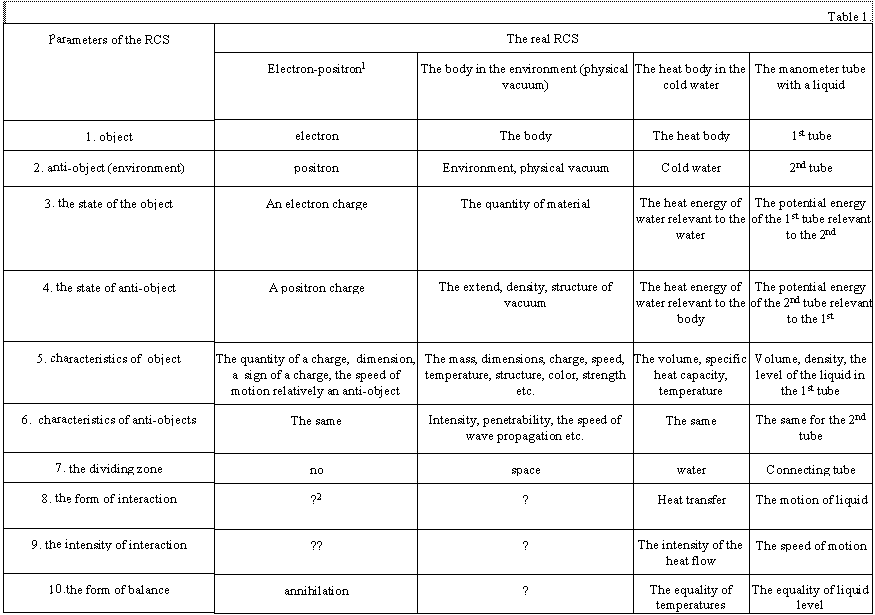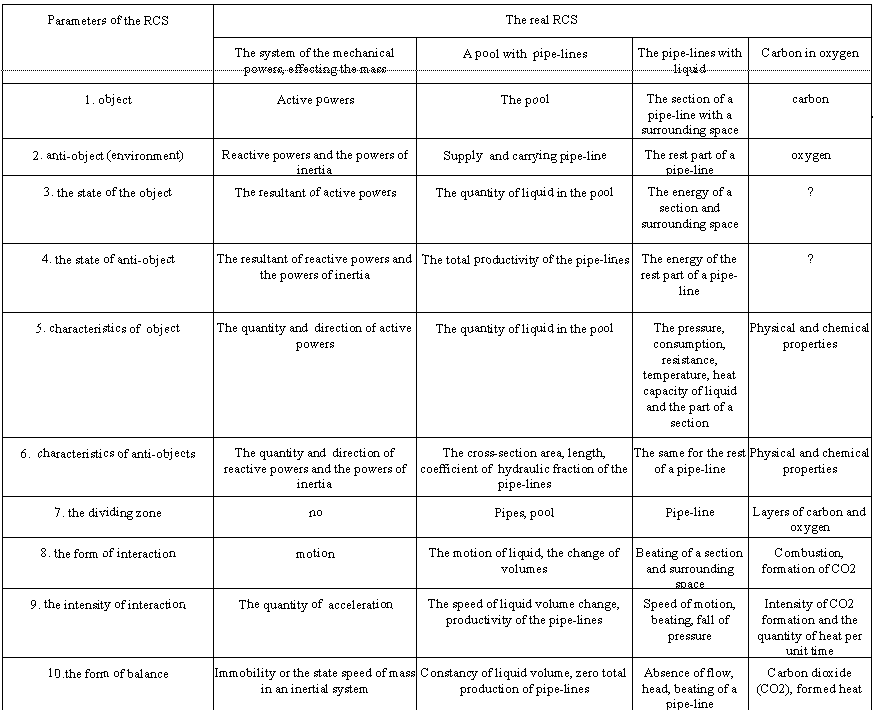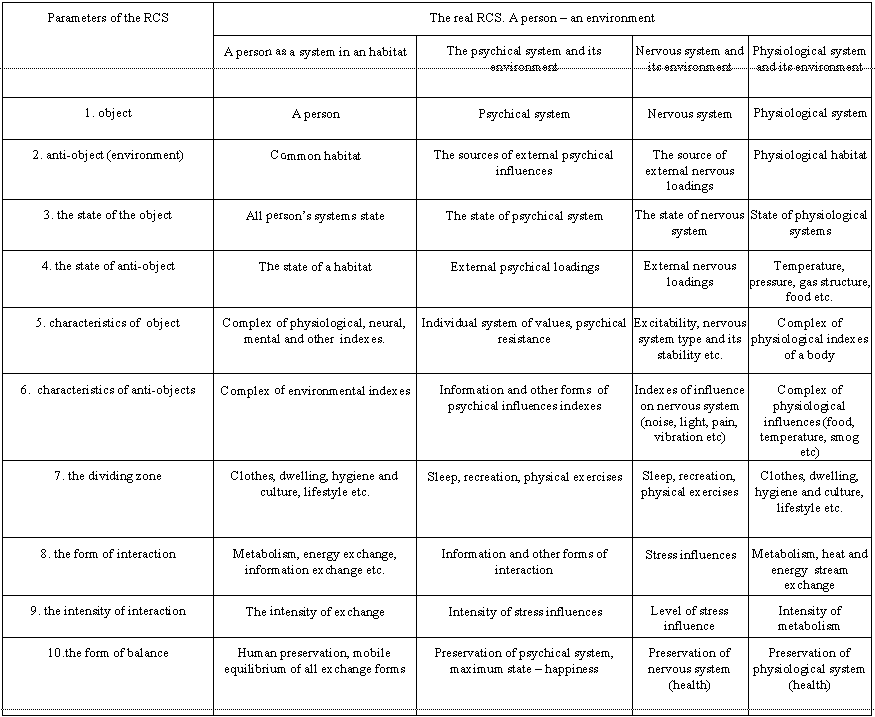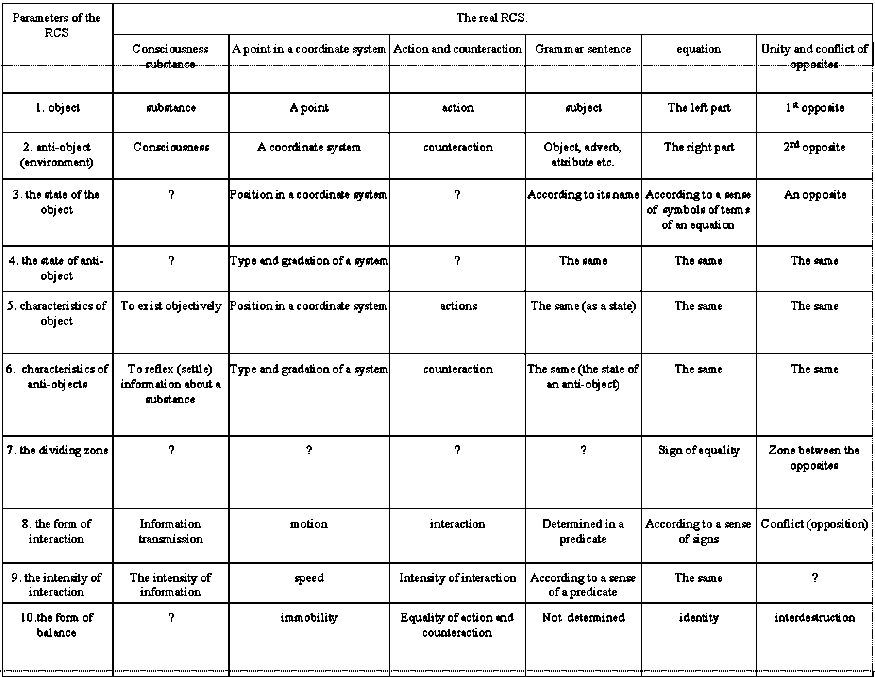If we add the sum of assumptions of all levels a CCS would be transformed into an ACS: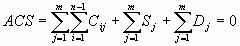= R(0) (13)

The dependences given above are describing CS only structurally not changing parts included in it, i.e. reflect only momentary state of a CS.